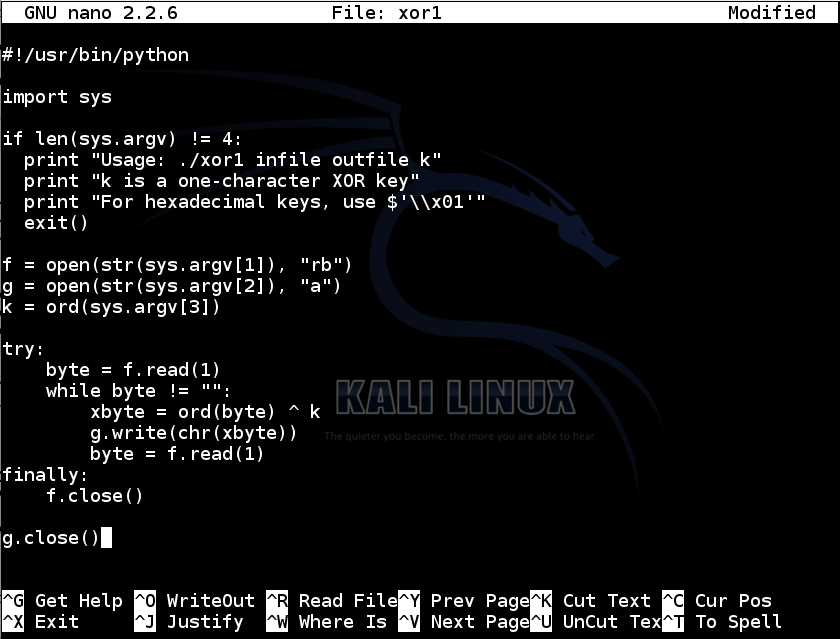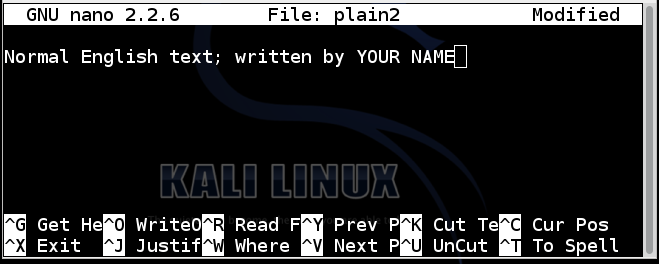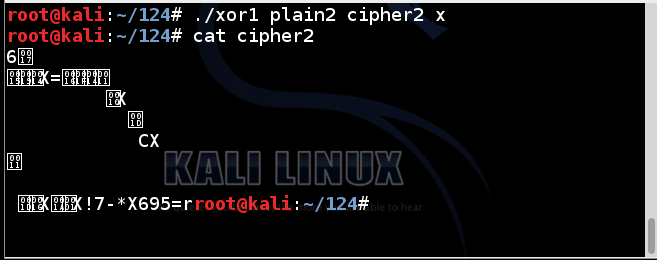# Proj 13: XOR Encryption in Python (10 pts.)

## What You Need

A Kali Linux machine, real or virtual. You could also use OS X, or Windows with Python installed.

## Purpose

Encrypt and decrypt files using XOR in Python.

## Understanding XOR

Exclusive OR (XOR) is a fundamental mathematical operation used in many encryption algorithms.

XOR operates on one bit at a time, with these results:

``` 0 XOR 0 = 0 0 XOR 1 = 1 1 XOR 0 = 1 1 XOR 1 = 0 ```
For our purposes, we'll use the Python ^ operator, which acts on a whole byte at a time.

Characters are ASCII-encoded, like this:

``` A is 01000001 B is 01000010 C is 01000011 ... ```
A whole table of ASCII values is here:

Consider A^B:

``` A is 01000001 B is 01000010 A^B= 00000011 ```
That is character 3, an unprintable end-of-text mark.

However, A^s is printable:

``` A is 01000001 s is 01110011 A^B= 00110010 ```
The result is the hexadecimal value 0x32, or the numeral 2.

## XOR in Python

In Kali Linux, in a Terminal window, execute this command:
```nano xor1 ```
In nano, enter the code shown below:
```#!/usr/bin/python import sys if len(sys.argv) != 4: print "Usage: ./xor1 infile outfile k" print "k is a one-character XOR key" print "For hexadecimal keys, use \$'\\x01'" exit() f = open(str(sys.argv), "rb") g = open(str(sys.argv), "a") k = ord(sys.argv) try: byte = f.read(1) while byte != "": xbyte = ord(byte) ^ k g.write(chr(xbyte)) byte = f.read(1) finally: f.close() g.close() ```Save the file with Ctrl+X, Y, Enter. Next, we need to make the file executable.

In a Terminal window, execute this command:

```chmod a+x xor1 ```

## Encrypting a Single Character

In a Terminal window, execute this command:
```./xor1 ```
You see the help message, explaining how to use the program, as shown below.To create a file named plain1 with the letter A in it, execute these commands :

```echo -n A > plain1 cat plain1 ```
The "echo -n" command created a file named plain1 which contains a single letter A, without a carriage return at the end of the file.

The "cat plain1" command printed out the file, which appeared as a single A at the start of the next line, as shown below:To encode the plain1 file with a key of s, execute these commands:

```./xor1 plain1 cipher1 s cat cipher1 ```
The result is 2, as shown below:## Encrypting a Text File

In Kali Linux, in a Terminal window, execute this command:
```nano plain2 ```
In nano, enter the code shown below, replacing "YOUR NAME" with your own name:
```Normal English text; written by YOUR NAME ```Save the file with Ctrl+X, Y, Enter. To encrypt the file using a key of x, execute these commands:

```./xor1 plain2 cipher2 x cat cipher2 ```
The result is strange unreadable characters, as shown below:## Decrypting a Text File

To decrypt a file, simply repeat the XOR operation with the same key. It will reverse itself.

Execute these commands:

```./xor1 cipher2 plain2r x cat plain2r ```
The file is restored to readable text, as shown below:## Capturing a Screen Image

Make sure YOUR NAME is visible, as shown above.

Click on the host system's taskbar, at the bottom of the screen.

Press the PrntScrn key to capture the whole desktop. Open Paint and paste in the image.

Save the image as "Proj 13 from YOUR NAME".

YOU MUST SEND IN A WHOLE-DESKTOP IMAGE FOR FULL CREDIT